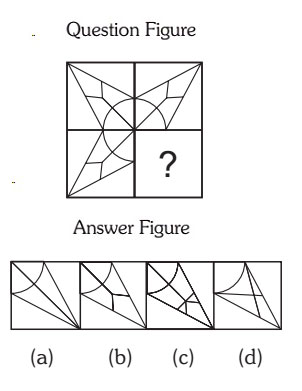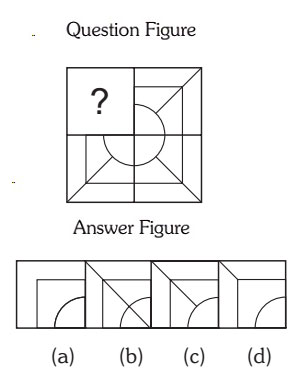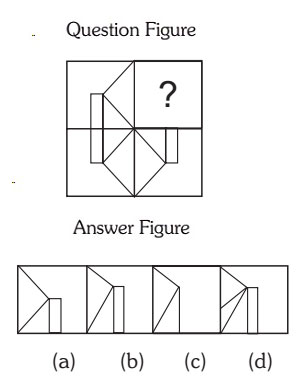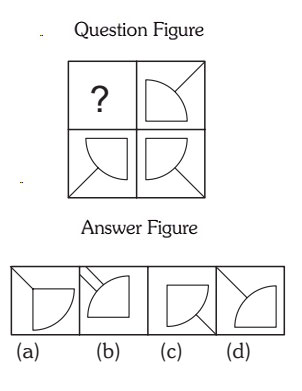## Completion Of Incomplete Pattern

#### Completion Of Incomplete Pattern

Direction: In each of the following questions, find out which of the answer figures (a), (b), (c) and (d) completes the figure?

1. Select a suitable figure from the four alternatives that would complete the figure.1. Select the figure which will complete the circle figure and arrow mark in the middle.

##### Correct Option: B

Option B figure will complete the circle and will show the correct arrow marks on the line also.

1. Select a suitable figure from the four alternatives that would complete the figure.1. By seeing the figure you need to complete the circle , square and rectangle with crossing the line from circle.

##### Correct Option: C

Answer C will complete the figure and will satisfy all the criteria.

1. Following Question has four alternatives, among which one completes the figures.1. Choose the figure which complete the rectangle and square.

##### Correct Option: B

Choose the figure which complete the rectangle and square. The answer figure (b) will complete the figure correctly.

1. Following Question has four alternatives, among which one completes the figures.1. See the figure carefully and you will find the option (d) will complete the figure.

##### Correct Option: D

See the figure carefully and you will find the option (d) will complete the figure.

1. Select the correct figure from the given Answer Figure that would complete the Question figure.1. The second and third figure are opposite to each other.

##### Correct Option: A

The second and third figure are opposite to each other. Hence 1 and 4th figure will be also opposite to each other.
Option (a) will be correct answer to complete the figure.